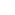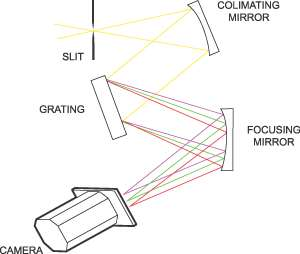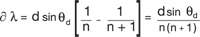Part of the Oxford Instruments Group
Expand

# Grating Orders and BlazingGratings can be designed to concentrate most of the incident intensity into a particular order, by a process called “blazing”. This is a process where the grooves of a grating are cut so that the reflecting surfaces are at a certain angle, the blaze angle. About 90% of the incident light is diffracted preferentially into the first order. Blazing works best when the incident beam and the diffracted beam fall equally on either side of the blaze angle.

When incident light is dispersed by a grating, more than one spectrum may be formed. These spectra or ‘orders’ can overlap Examination of the grating equation shows that the diffracted angle of a wavelength of light l1 in the 1st order will be the same angle or direction for the wavelength l2 in the second order where l2 = l1/2 . These higher orders could cause confusion when measuring a spectrum of light. In this case, order sorting filters should be used to block the second order light and thereby reduce errors in the measurement of the spectra.

## The Free Spectral Range

Simply stated, the free spectral range of a spectrometer is the wavelength interval, ∂λ, for which there is a single-valued relationship between wavelength λ and the position x(λ) of the entrance slit image. For prisms, the free spectral range usually covers the whole region of dispersion of the prism (and is determined by the nature of the material used) whilst for grating spectrometers the free spectral range is determined by the grating diffraction order n. So for grating spectrometers, the free spectral range is the range of wavelengths in which there is no interference or superposition of light from adjacent orders.Using the grating equation we can show for light incident vertically ( θi = 90°) and wavelength λ will be diffracted at a angle θd so that λ1 = d sin θd . A second wavelength λ2 in a higher order (n+1) will also be diffracted in the same direction angle if (n+1)λ2 = d sinθd , Hence the free spectral range, therefore, can be expressed as:Thus the free spectral range decreases with increasing diffraction order, and applies to all gratings capable of operating in more than one order. It becomes an important factor with specialized gratings, such as Echelle gratings, that function at very high orders, as well as with many interferometers. All these devices exhibit very limited (small) free spectral ranges.

## Czerny-Turner Spectrograph

There are many arrangements of spectrographs using plane gratings but a representative one is the Czerny-Turner spectrograph as shown in the diagram above.A spectrograph typically contains two types of optics. The first forms an image of the source, the second disperses the light or makes an angular deflection of the light as a function of the wavelength. Together they make an instrument which forms multiple images of the source, one for each of the separate resolved wavelengths.In the Czerny-Turner spectrograph the imaging optics use a pair of concave mirrors and the dispersive element is a plane grating. The first mirror collimates the light from the slit and directs it on the grating. The second mirror gathers the light from the grating and directs the multiple images of the input slit onto the detector. The concave mirrors are carefully arranged to minimize the optical aberrations introduced by the mirrors. The range of wavelengths detected can be varied by rotating the plane grating angle to select the required diffracted images.The off angle mirrors do introduce an astigmatism aberration which takes an image of a point and stretches it into a line. For regular spectroscopy this is not usually a problem however if the experimenter is imaging multiple fibres or is imaging a line the astigmatism can degrade the effective spatial resolution or cause multiple spectra from individual fibres to overlap. In this case an imaging spectrograph needs to be used.An imaging spectrograph removes the astigmatism in one of two ways. The spectrograph can either use specially designed mirrors called toroidial mirrors to correct the astigmatism or use correction optics. Toroidial mirrors have different focal lengths.

Date: N/A

Author: Andor

Category: Technical Article